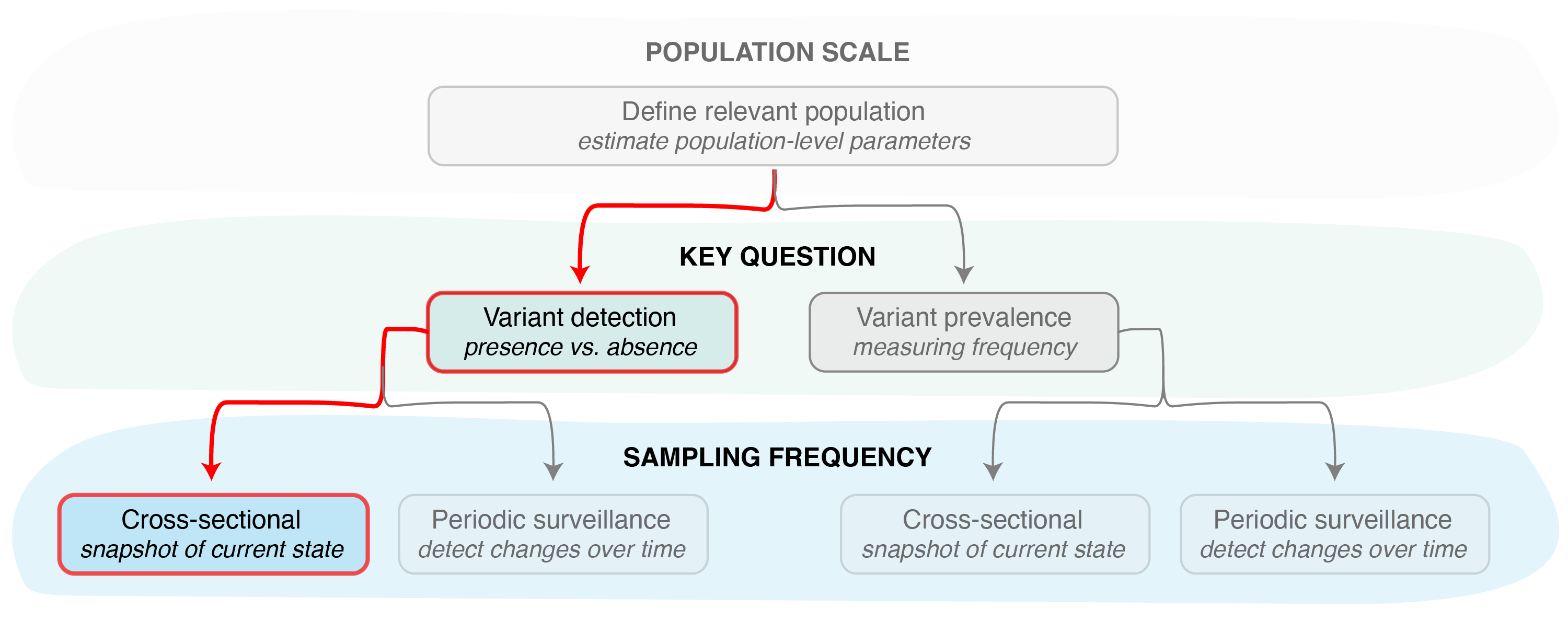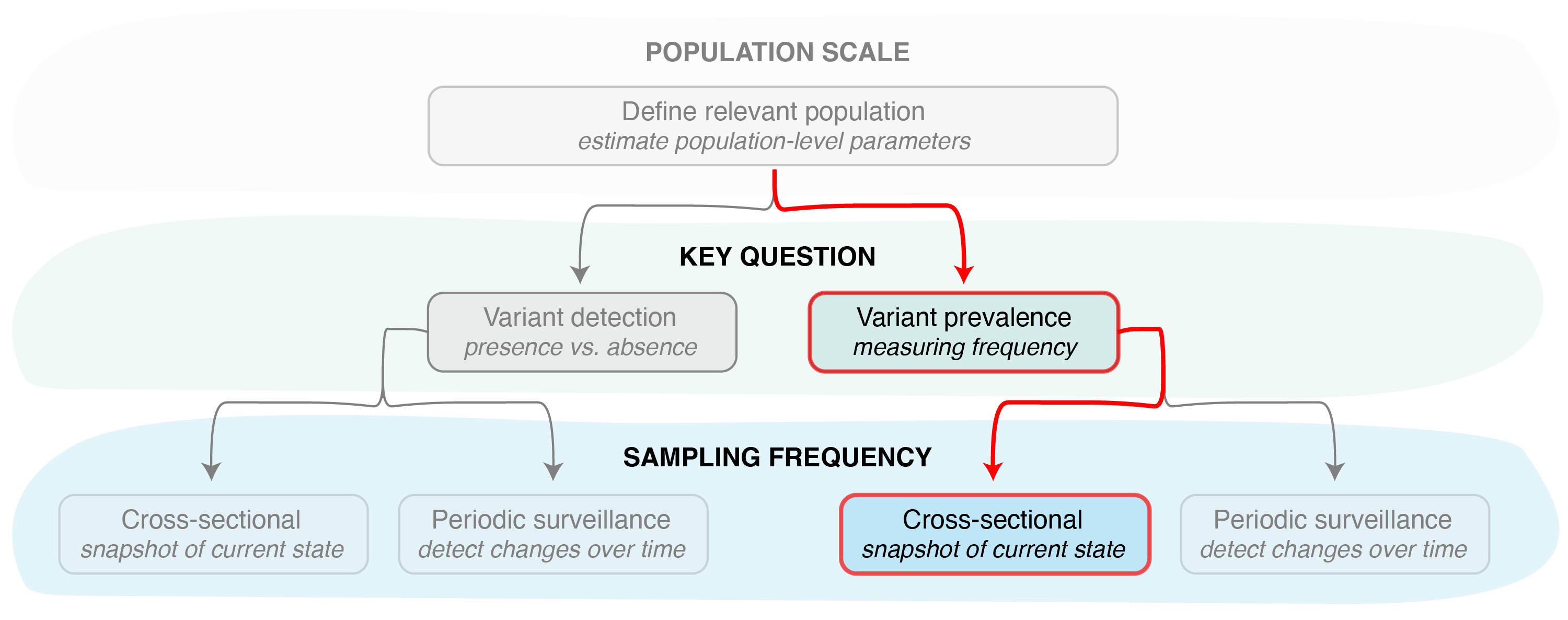# Estimating the sample size needed for variant monitoring

### Cross-sectional sampling

This vignette provides an overview of the functions that can be used to estimate the sample size needed to detect a pathogen variant or estimate its frequency in a population, given molecular characterization of a single cross-sectional sample of pathogen infections.

##### Variant detectionFigure 1. Identifying the key question (detection vs prevalence monitoring) is the second step in designing a pathogen surveillance system. Determining the sample size needed to answer this question then requires determining the sampling frequency, i.e., if samples will be collected and sequenced just once (cross-sectional sampling) or with some regularity over a period of time (periodic surveillance).

When the goal is detecting the presence or absence of a specific variant in a population using a single cross-sectional sample (Figure 1), we can use the vartrack_samplesize_detect() function with the sampling_freq = "xsect" option. Applying this function, however, requires knowledge of the coefficient of detection ratio between two pathogen variants (or, more commonly, one variant and the rest of the pathogen population). The coefficient of detection ratio for two variants can be calculated using the vartrack_cod_ratio() function (see Estimating bias in observed variant prevalence for more details). Since we are only interested in the ratio of the coefficients of detection, applying this function only requires providing parameters which are expected to differ between variants. The ratio between any variants not provided is assumed to be equal to one.

Once we have an estimate of the coefficient of detection ratio, we can calculate the sample size needed for detection from the following parameters:

Param Variable Name Description
$$P_{V_1}$$ p_v1 the desired minimum variant prevalence to be able to detect
$$p$$ prob the desired probability of detection
$$\omega$$ omega the sequencing success rate
$$\frac{C_{V_1}}{C_{V_2}}$$ c_ratio the coefficient of detection ratio, calculated as the ratio of the coefficients of variant 1 to variant 2 (can be calculated using vartrack_cod_ratio())

We then apply this sample size calculation function as follows:

library(phylosamp)
vartrack_samplesize_detect(p_v1=0.02, prob=0.95, omega=0.8,
c_ratio=1.368, sampling_freq="xsect")
## Calculating sample size for variant detection assuming single cross-sectional sample
##  135.9928

In other words, 136 samples are needed to detect a variant at 2% (or higher) in a population with 95% of detection, given a coefficient of detection ratio ($$\frac{C_{V_1}}{C_{V_2}}$$) of 1.368 and a single, cross-sectional sample. This takes into account the fact that not all samples sequenced (or otherwise characterized) will be successful. We assume an 80% success rate ($$\omega=0.8$$), which ensures a selection of 136 samples will produce the 109 high quality data points that can be used to detect the presence of a pathogen variant.

##### Variant prevalenceFigure 2. Other functions in the phylosamp package can be used to determine the sample size needed to accurately monitor variant prevalence, again assuming a single cross-sectional sample.

In some cases, it may not be enough to simply detect a variant—we may want to estimate its frequency in the population (Figure 2). In that case, we can use the vartrack_samplesize_prev() function to determine the sample size needed to estimate the prevalence of a variant in a population given some desired precision. This function requires the user to specific a slightly different set of parameters:

Param Variable Name Description
$$P_{V_1}$$ p_v1 the desired minimum variant prevalence to be able to detect
$$p$$ prob the desired probability of detection
$$d$$ precision the desired precision in the prevalence estimate
$$\omega$$ omega the sequencing success rate
$$\frac{C_{V_1}}{C_{V_2}}$$ c_ratio the coefficient of detection ratio, calculated as the ratio of the coefficients of variant 1 to variant 2 (can be calculated using vartrack_cod_ratio())

We then can calculate sample size as follows:

c1_c2 <- vartrack_cod_ratio(psi_v1=0.25, psi_v2=0.4, tau_a=0.05, tau_s=0.3)
vartrack_samplesize_prev(p_v1=0.1,prob=0.95,precision=0.25,
omega=0.8, c_ratio=c1_c2, sampling_freq="xsect")
## Calculating sample size for variant prevalence estimation assuming single cross-sectional sample
##  582.2843

In other words, 583 samples must be processed in order to estimate the population prevalence of a variant with at least 10% prevalence in the population, with a precision of 25% of the true value, and a confidence of 95% in the prevalence estimate. Again, we assume an 80% success rate, which ensures successful characterization of 466 of the 583 samples selected for sequencing so they can be used to detect the presence of a pathogen variant.

##### Other sampling scenarios

For information on functions that can be used to estimate the sample size given a periodic sampling approach, see Estimating the sample size needed for variant monitoring: periodic sampling. These functions can also be used in “reverse”, to calculate the probability of detection given some sampling scheme, as in the Estimating the probability of detecting a variant cross-sectional and periodic vignettes.